Anúncio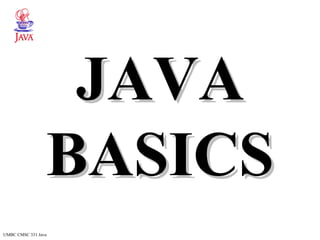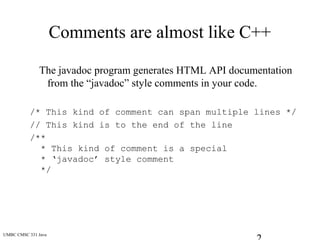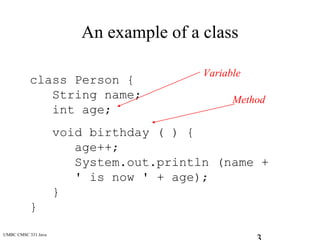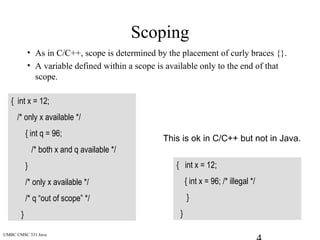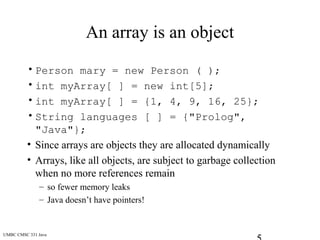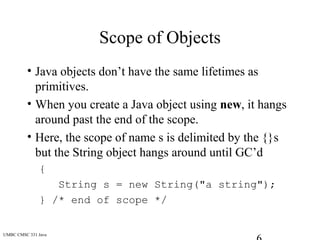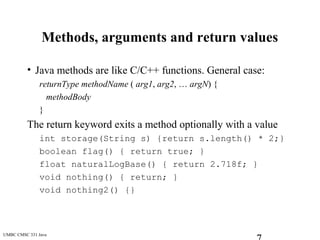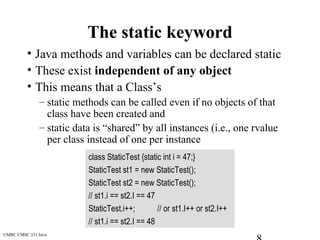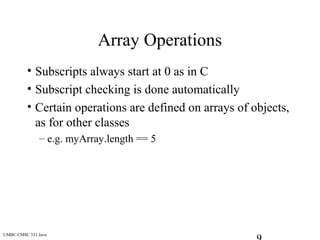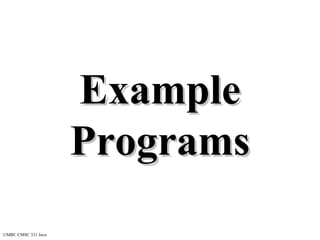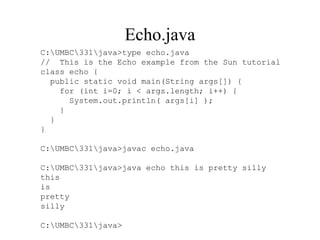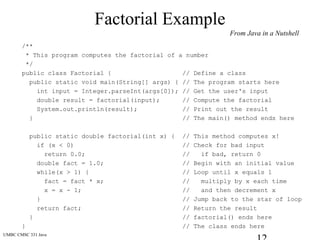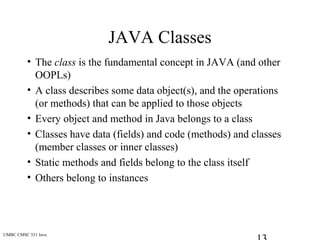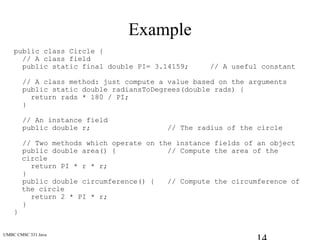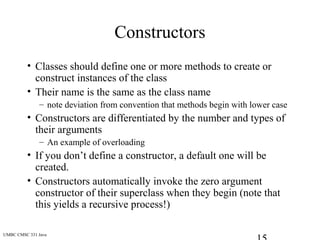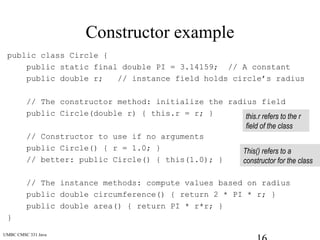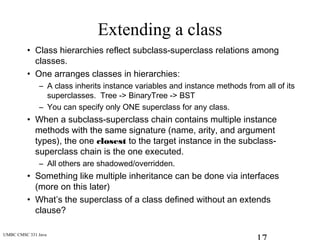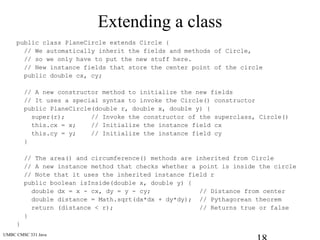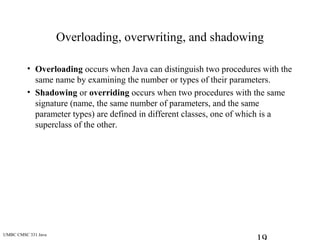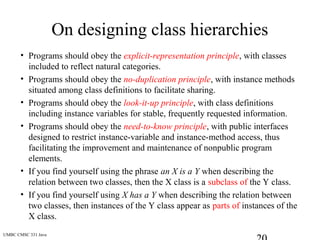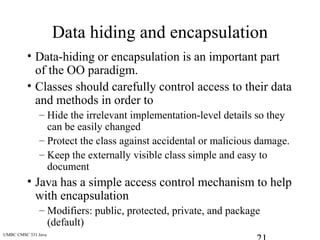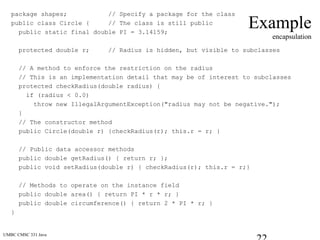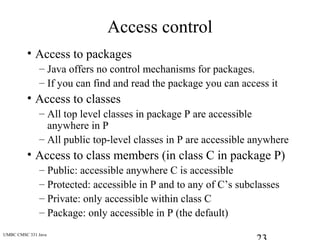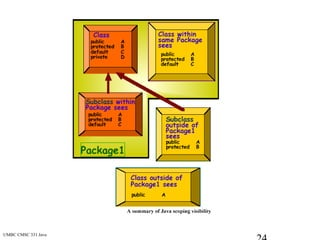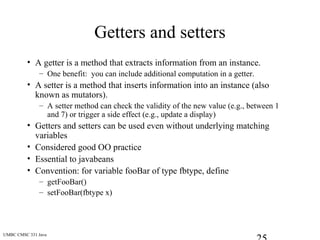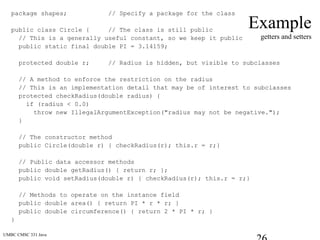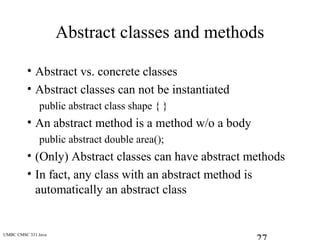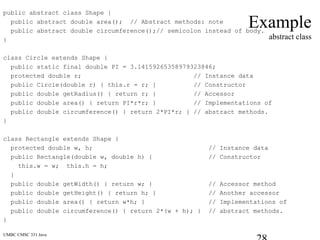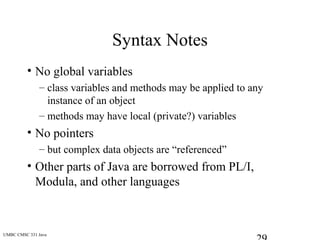1 de 29
Anúncio

### Java basic

1. UMBC CMSC 331 Java JAVAJAVA BASICSBASICS
2. UMBC CMSC 331 Java Comments are almost like C++ The javadoc program generates HTML API documentation from the “javadoc” style comments in your code. /* This kind of comment can span multiple lines */ // This kind is to the end of the line /** * This kind of comment is a special * ‘javadoc’ style comment */
3. UMBC CMSC 331 Java An example of a class class Person { String name; int age; void birthday ( ) { age++; System.out.println (name + ' is now ' + age); } } Variable Method
4. UMBC CMSC 331 Java Scoping • As in C/C++, scope is determined by the placement of curly braces {}. • A variable defined within a scope is available only to the end of that scope. { int x = 12; /* only x available */ { int q = 96; /* both x and q available */ } /* only x available */ /* q “out of scope” */ } { int x = 12; { int x = 96; /* illegal */ } } This is ok in C/C++ but not in Java.
5. UMBC CMSC 331 Java An array is an object • Person mary = new Person ( ); • int myArray[ ] = new int; • int myArray[ ] = {1, 4, 9, 16, 25}; • String languages [ ] = {"Prolog", "Java"}; • Since arrays are objects they are allocated dynamically • Arrays, like all objects, are subject to garbage collection when no more references remain – so fewer memory leaks – Java doesn’t have pointers!
6. UMBC CMSC 331 Java Scope of Objects • Java objects don’t have the same lifetimes as primitives. • When you create a Java object using new, it hangs around past the end of the scope. • Here, the scope of name s is delimited by the {}s but the String object hangs around until GC’d { String s = new String("a string"); } /* end of scope */
7. UMBC CMSC 331 Java Methods, arguments and return values • Java methods are like C/C++ functions. General case: returnType methodName ( arg1, arg2, … argN) { methodBody } The return keyword exits a method optionally with a value int storage(String s) {return s.length() * 2;} boolean flag() { return true; } float naturalLogBase() { return 2.718f; } void nothing() { return; } void nothing2() {}
8. UMBC CMSC 331 Java The static keyword • Java methods and variables can be declared static • These exist independent of any object • This means that a Class’s – static methods can be called even if no objects of that class have been created and – static data is “shared” by all instances (i.e., one rvalue per class instead of one per instance class StaticTest {static int i = 47;} StaticTest st1 = new StaticTest(); StaticTest st2 = new StaticTest(); // st1.i == st2.I == 47 StaticTest.i++; // or st1.I++ or st2.I++ // st1.i == st2.I == 48
9. UMBC CMSC 331 Java Array Operations • Subscripts always start at 0 as in C • Subscript checking is done automatically • Certain operations are defined on arrays of objects, as for other classes – e.g. myArray.length == 5
10. UMBC CMSC 331 Java ExampleExample ProgramsPrograms
11. Echo.java C:UMBC331java>type echo.java // This is the Echo example from the Sun tutorial class echo { public static void main(String args[]) { for (int i=0; i < args.length; i++) { System.out.println( args[i] ); } } } C:UMBC331java>javac echo.java C:UMBC331java>java echo this is pretty silly this is pretty silly C:UMBC331java>
12. UMBC CMSC 331 Java Factorial Example /** * This program computes the factorial of a number */ public class Factorial { // Define a class public static void main(String[] args) { // The program starts here int input = Integer.parseInt(args); // Get the user's input double result = factorial(input); // Compute the factorial System.out.println(result); // Print out the result } // The main() method ends here public static double factorial(int x) { // This method computes x! if (x < 0) // Check for bad input return 0.0; // if bad, return 0 double fact = 1.0; // Begin with an initial value while(x > 1) { // Loop until x equals 1 fact = fact * x; // multiply by x each time x = x - 1; // and then decrement x } // Jump back to the star of loop return fact; // Return the result } // factorial() ends here } // The class ends here From Java in a Nutshell
13. UMBC CMSC 331 Java JAVA Classes • The class is the fundamental concept in JAVA (and other OOPLs) • A class describes some data object(s), and the operations (or methods) that can be applied to those objects • Every object and method in Java belongs to a class • Classes have data (fields) and code (methods) and classes (member classes or inner classes) • Static methods and fields belong to the class itself • Others belong to instances
14. UMBC CMSC 331 Java Example public class Circle { // A class field public static final double PI= 3.14159; // A useful constant // A class method: just compute a value based on the arguments public static double radiansToDegrees(double rads) { return rads * 180 / PI; } // An instance field public double r; // The radius of the circle // Two methods which operate on the instance fields of an object public double area() { // Compute the area of the circle return PI * r * r; } public double circumference() { // Compute the circumference of the circle return 2 * PI * r; } }
15. UMBC CMSC 331 Java Constructors • Classes should define one or more methods to create or construct instances of the class • Their name is the same as the class name – note deviation from convention that methods begin with lower case • Constructors are differentiated by the number and types of their arguments – An example of overloading • If you don’t define a constructor, a default one will be created. • Constructors automatically invoke the zero argument constructor of their superclass when they begin (note that this yields a recursive process!)
16. UMBC CMSC 331 Java Constructor example public class Circle { public static final double PI = 3.14159; // A constant public double r; // instance field holds circle’s radius // The constructor method: initialize the radius field public Circle(double r) { this.r = r; } // Constructor to use if no arguments public Circle() { r = 1.0; } // better: public Circle() { this(1.0); } // The instance methods: compute values based on radius public double circumference() { return 2 * PI * r; } public double area() { return PI * r*r; } } this.r refers to the r field of the class This() refers to a constructor for the class
17. UMBC CMSC 331 Java Extending a class • Class hierarchies reflect subclass-superclass relations among classes. • One arranges classes in hierarchies: – A class inherits instance variables and instance methods from all of its superclasses. Tree -> BinaryTree -> BST – You can specify only ONE superclass for any class. • When a subclass-superclass chain contains multiple instance methods with the same signature (name, arity, and argument types), the one closest to the target instance in the subclass- superclass chain is the one executed. – All others are shadowed/overridden. • Something like multiple inheritance can be done via interfaces (more on this later) • What’s the superclass of a class defined without an extends clause?
18. UMBC CMSC 331 Java Extending a class public class PlaneCircle extends Circle { // We automatically inherit the fields and methods of Circle, // so we only have to put the new stuff here. // New instance fields that store the center point of the circle public double cx, cy; // A new constructor method to initialize the new fields // It uses a special syntax to invoke the Circle() constructor public PlaneCircle(double r, double x, double y) { super(r); // Invoke the constructor of the superclass, Circle() this.cx = x; // Initialize the instance field cx this.cy = y; // Initialize the instance field cy } // The area() and circumference() methods are inherited from Circle // A new instance method that checks whether a point is inside the circle // Note that it uses the inherited instance field r public boolean isInside(double x, double y) { double dx = x - cx, dy = y - cy; // Distance from center double distance = Math.sqrt(dx*dx + dy*dy); // Pythagorean theorem return (distance < r); // Returns true or false } }
19. UMBC CMSC 331 Java Overloading, overwriting, and shadowing • Overloading occurs when Java can distinguish two procedures with the same name by examining the number or types of their parameters. • Shadowing or overriding occurs when two procedures with the same signature (name, the same number of parameters, and the same parameter types) are defined in different classes, one of which is a superclass of the other.
20. UMBC CMSC 331 Java On designing class hierarchies • Programs should obey the explicit-representation principle, with classes included to reflect natural categories. • Programs should obey the no-duplication principle, with instance methods situated among class definitions to facilitate sharing. • Programs should obey the look-it-up principle, with class definitions including instance variables for stable, frequently requested information. • Programs should obey the need-to-know principle, with public interfaces designed to restrict instance-variable and instance-method access, thus facilitating the improvement and maintenance of nonpublic program elements. • If you find yourself using the phrase an X is a Y when describing the relation between two classes, then the X class is a subclass of the Y class. • If you find yourself using X has a Y when describing the relation between two classes, then instances of the Y class appear as parts of instances of the X class.
21. UMBC CMSC 331 Java Data hiding and encapsulation • Data-hiding or encapsulation is an important part of the OO paradigm. • Classes should carefully control access to their data and methods in order to – Hide the irrelevant implementation-level details so they can be easily changed – Protect the class against accidental or malicious damage. – Keep the externally visible class simple and easy to document • Java has a simple access control mechanism to help with encapsulation – Modifiers: public, protected, private, and package (default)
22. UMBC CMSC 331 Java Example encapsulation package shapes; // Specify a package for the class public class Circle { // The class is still public public static final double PI = 3.14159; protected double r; // Radius is hidden, but visible to subclasses // A method to enforce the restriction on the radius // This is an implementation detail that may be of interest to subclasses protected checkRadius(double radius) { if (radius < 0.0) throw new IllegalArgumentException("radius may not be negative."); } // The constructor method public Circle(double r) {checkRadius(r); this.r = r; } // Public data accessor methods public double getRadius() { return r; }; public void setRadius(double r) { checkRadius(r); this.r = r;} // Methods to operate on the instance field public double area() { return PI * r * r; } public double circumference() { return 2 * PI * r; } }
23. UMBC CMSC 331 Java Access control • Access to packages – Java offers no control mechanisms for packages. – If you can find and read the package you can access it • Access to classes – All top level classes in package P are accessible anywhere in P – All public top-level classes in P are accessible anywhere • Access to class members (in class C in package P) – Public: accessible anywhere C is accessible – Protected: accessible in P and to any of C’s subclasses – Private: only accessible within class C – Package: only accessible in P (the default)
24. UMBC CMSC 331 Java
25. UMBC CMSC 331 Java Getters and setters • A getter is a method that extracts information from an instance. – One benefit: you can include additional computation in a getter. • A setter is a method that inserts information into an instance (also known as mutators). – A setter method can check the validity of the new value (e.g., between 1 and 7) or trigger a side effect (e.g., update a display) • Getters and setters can be used even without underlying matching variables • Considered good OO practice • Essential to javabeans • Convention: for variable fooBar of type fbtype, define – getFooBar() – setFooBar(fbtype x)
26. UMBC CMSC 331 Java Example getters and setters package shapes; // Specify a package for the class public class Circle { // The class is still public // This is a generally useful constant, so we keep it public public static final double PI = 3.14159; protected double r; // Radius is hidden, but visible to subclasses // A method to enforce the restriction on the radius // This is an implementation detail that may be of interest to subclasses protected checkRadius(double radius) { if (radius < 0.0) throw new IllegalArgumentException("radius may not be negative."); } // The constructor method public Circle(double r) { checkRadius(r); this.r = r;} // Public data accessor methods public double getRadius() { return r; }; public void setRadius(double r) { checkRadius(r); this.r = r;} // Methods to operate on the instance field public double area() { return PI * r * r; } public double circumference() { return 2 * PI * r; } }
27. UMBC CMSC 331 Java Abstract classes and methods • Abstract vs. concrete classes • Abstract classes can not be instantiated public abstract class shape { } • An abstract method is a method w/o a body public abstract double area(); • (Only) Abstract classes can have abstract methods • In fact, any class with an abstract method is automatically an abstract class
28. UMBC CMSC 331 Java Example abstract class public abstract class Shape { public abstract double area(); // Abstract methods: note public abstract double circumference();// semicolon instead of body. } class Circle extends Shape { public static final double PI = 3.14159265358979323846; protected double r; // Instance data public Circle(double r) { this.r = r; } // Constructor public double getRadius() { return r; } // Accessor public double area() { return PI*r*r; } // Implementations of public double circumference() { return 2*PI*r; } // abstract methods. } class Rectangle extends Shape { protected double w, h; // Instance data public Rectangle(double w, double h) { // Constructor this.w = w; this.h = h; } public double getWidth() { return w; } // Accessor method public double getHeight() { return h; } // Another accessor public double area() { return w*h; } // Implementations of public double circumference() { return 2*(w + h); } // abstract methods. }
29. UMBC CMSC 331 Java Syntax Notes • No global variables – class variables and methods may be applied to any instance of an object – methods may have local (private?) variables • No pointers – but complex data objects are “referenced” • Other parts of Java are borrowed from PL/I, Modula, and other languages
Anúncio